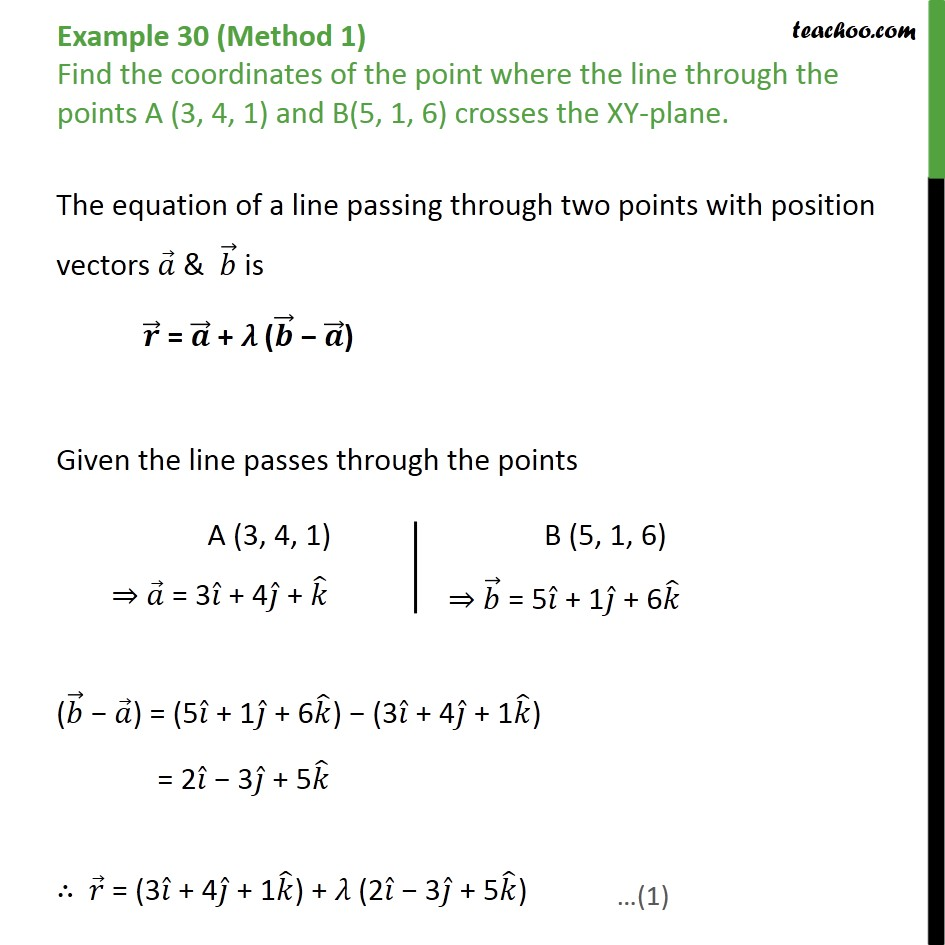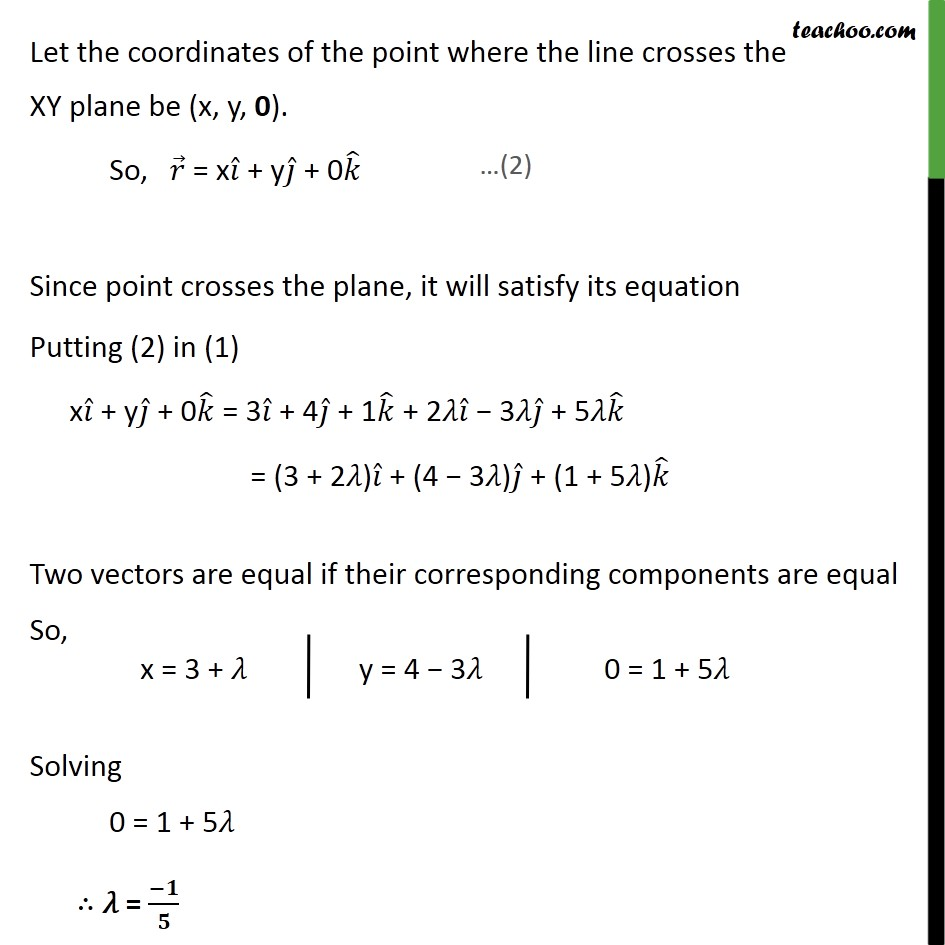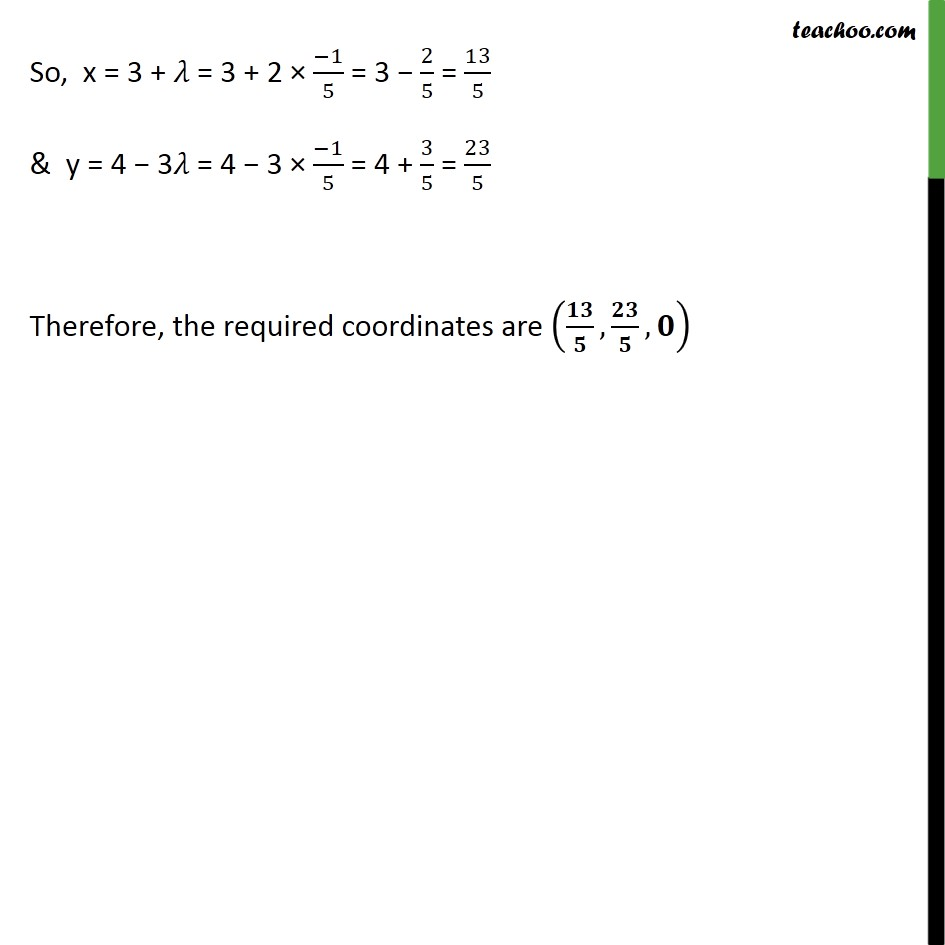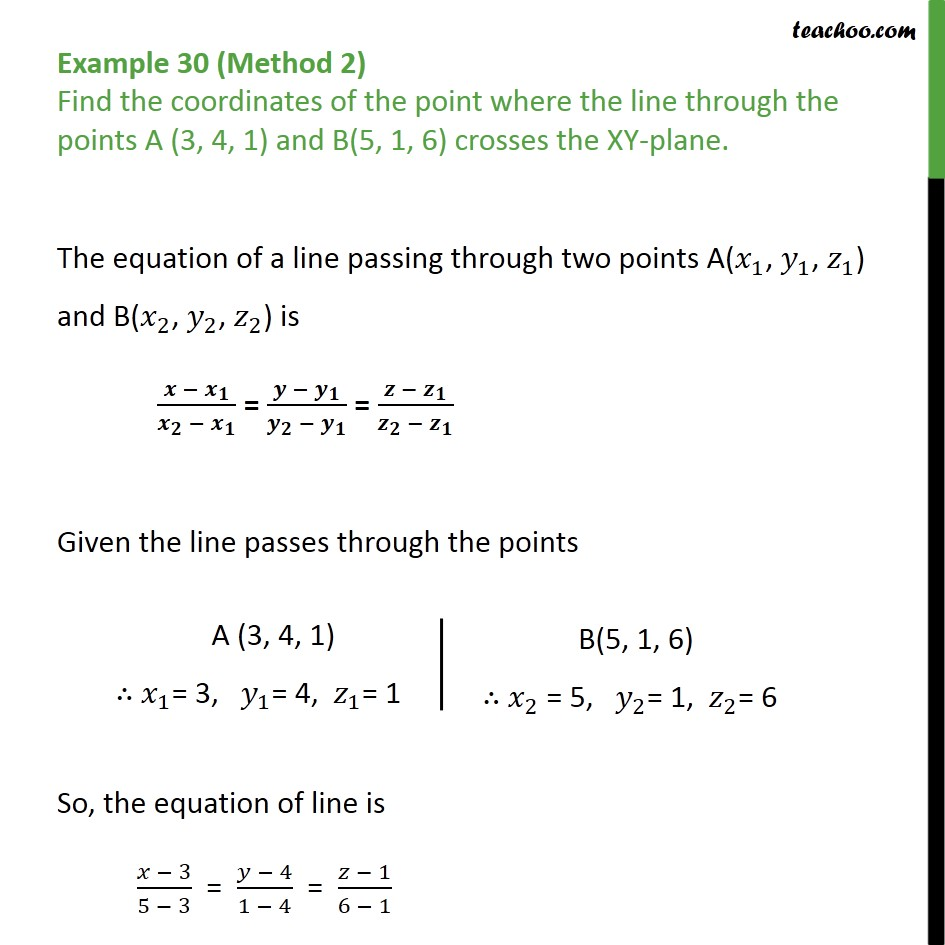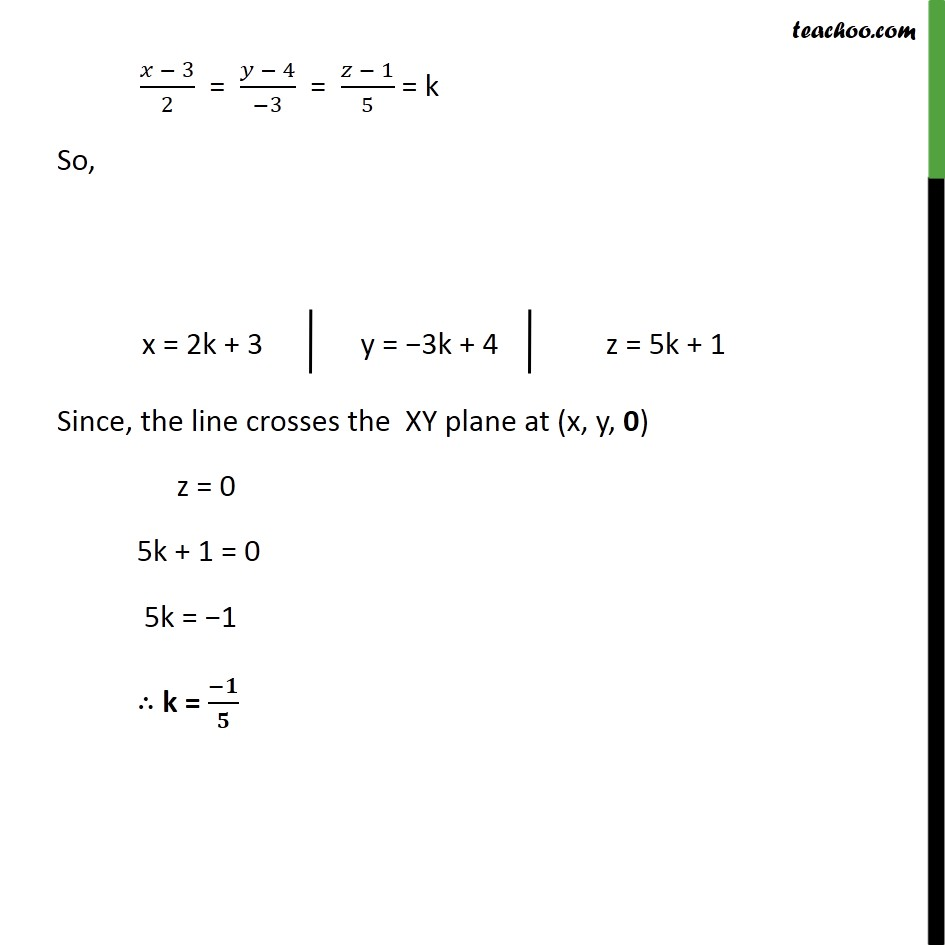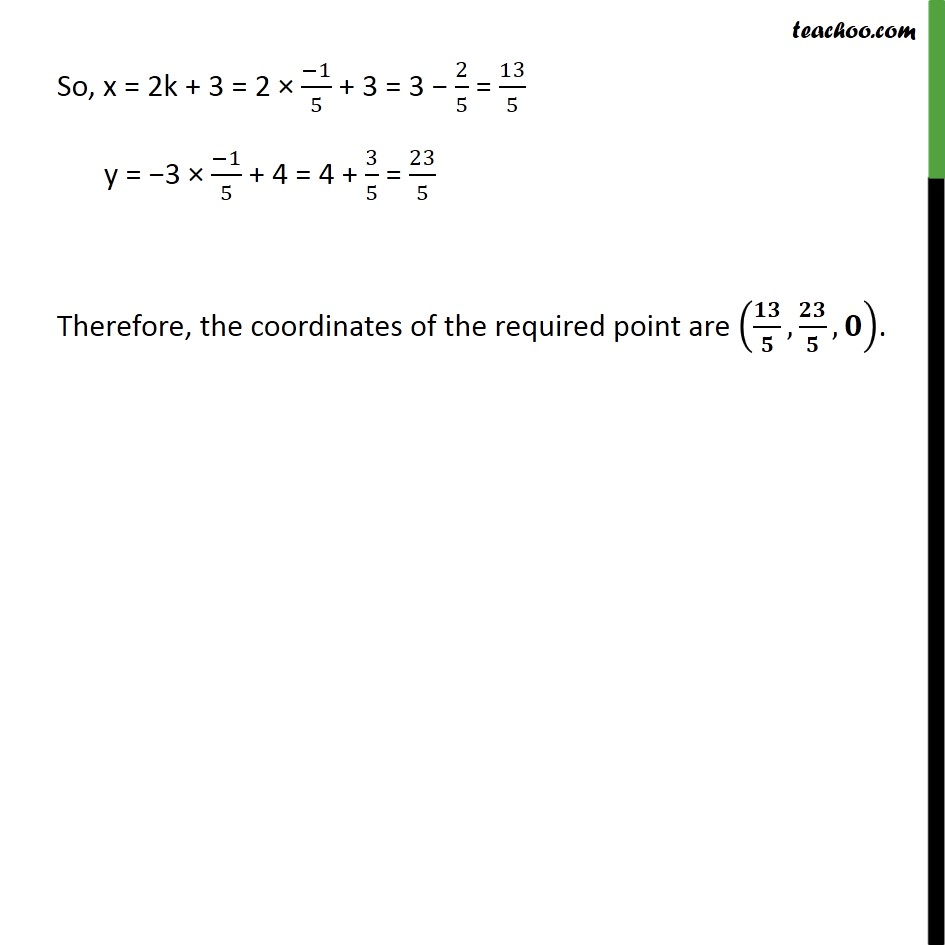1. Chapter 11 Class 12 Three Dimensional Geometry
2. Concept wise
3. Point with Lines and Planes

Transcript

Example 30 (Method 1) Find the coordinates of the point where the line through the points A (3, 4, 1) and B(5, 1, 6) crosses the XY-plane. The equation of a line passing through two points with position vectors 𝑎﷯ & 𝑏﷯ is 𝒓﷯ = 𝒂﷯ + 𝜆 ( 𝒃﷯ − 𝒂﷯) Given the line passes through the points ( 𝑏﷯ − 𝑎﷯) = (5 𝑖﷯ + 1 𝑗﷯ + 6 𝑘﷯) − (3 𝑖﷯ + 4 𝑗﷯ + 1 𝑘﷯) = 2 𝑖﷯ − 3 𝑗﷯ + 5 𝑘﷯ ∴ 𝑟﷯ = (3 𝑖﷯ + 4 𝑗﷯ + 1 𝑘﷯) + 𝜆 (2 𝑖﷯ − 3 𝑗﷯ + 5 𝑘﷯) Let the coordinates of the point where the line crosses the XY plane be (x, y, 0). So, 𝑟﷯ = x 𝑖﷯ + y 𝑗﷯ + 0 𝑘﷯ Since point crosses the plane, it will satisfy its equation Putting (2) in (1) x 𝑖﷯ + y 𝑗﷯ + 0 𝑘﷯ = 3 𝑖﷯ + 4 𝑗﷯ + 1 𝑘﷯ + 2𝜆 𝑖﷯ − 3𝜆 𝑗﷯ + 5𝜆 𝑘﷯ = (3 + 2𝜆) 𝑖﷯ + (4 − 3𝜆) 𝑗﷯ + (1 + 5𝜆) 𝑘﷯ Two vectors are equal if their corresponding components are equal So, Solving 0 = 1 + 5𝜆 ∴ 𝜆 = −𝟏﷮𝟓﷯ So, x = 3 + 𝜆 = 3 + 2 × −1﷮5﷯ = 3 − 2﷮5﷯ = 13﷮5﷯ & y = 4 − 3𝜆 = 4 − 3 × −1﷮5﷯ = 4 + 3﷮5﷯ = 23﷮5﷯ Therefore, the required coordinates are 𝟏𝟑﷮𝟓﷯, 𝟐𝟑﷮𝟓﷯,𝟎﷯ Example 30 (Method 2) Find the coordinates of the point where the line through the points A (3, 4, 1) and B(5, 1, 6) crosses the XY-plane. The equation of a line passing through two points A( 𝑥﷮1﷯, 𝑦﷮1﷯, 𝑧﷮1﷯) and B( 𝑥﷮2﷯, 𝑦﷮2﷯, 𝑧﷮2﷯) is 𝒙 − 𝒙﷮𝟏﷯﷮ 𝒙﷮𝟐﷯ − 𝒙﷮𝟏﷯﷯ = 𝒚 − 𝒚﷮𝟏﷯﷮ 𝒚﷮𝟐﷯ − 𝒚﷮𝟏﷯﷯ = 𝒛 − 𝒛﷮𝟏﷯﷮ 𝒛﷮𝟐﷯ − 𝒛﷮𝟏﷯﷯ Given the line passes through the points So, the equation of line is 𝑥 − 3﷮5 − 3﷯ = 𝑦 − 4﷮1 − 4﷯ = 𝑧 − 1﷮6 − 1﷯ 𝑥 − 3﷮2﷯ = 𝑦 − 4﷮−3﷯ = 𝑧 − 1﷮5﷯ = k So, Since, the line crosses the XY plane at (x, y, 0) z = 0 5k + 1 = 0 5k = −1 ∴ k = −𝟏﷮𝟓﷯ So, x = 2k + 3 = 2 × −1﷮5﷯ + 3 = 3 − 2﷮5﷯ = 13﷮5﷯ y = −3 × −1﷮5﷯ + 4 = 4 + 3﷮5﷯ = 23﷮5﷯ Therefore, the coordinates of the required point are 𝟏𝟑﷮𝟓﷯, 𝟐𝟑﷮𝟓﷯, 𝟎﷯.

Point with Lines and Planes

About the AuthorDavneet Singh
Davneet Singh is a graduate from Indian Institute of Technology, Kanpur. He has been teaching from the past 9 years. He provides courses for Maths and Science at Teachoo.